3 November 2019

# Make and Put a Cell

### How make cells and put them in a layout

(For using polygons in a cell see also Create a building block using Polygons)

#### The Nazca cell

Layout structures in Nazca are grouped in “cells”, very much alike a gds cell hierarchy. A cell can contain the following Nazca mask objects:

• – Cells
• – Polygons
• – Polylines
• – Annotations
• – Pins

Each Nazca mask object has a “put” method to place it in a Nazca cell. Note that as soon as nazca is imported with `import nazca as nd`  it a creates and activates a cell named “nazca” for you, so you do not have to create the first Cell. Below is a code example that shows how to place a straight and bent waveguide and export it to gds. The result is shown in the figure on the right. Hence, the structures in gds in this case are placed in the cell named “nazca”. Note that an empty put() positions the element at the “current pin” or “cp”. For the first element in a new cell this is position (x, y, a) = (0, 0, 0). After an element has been placed (put), the cp is set to be the default output pin of that element, so placing components sequentially with put() connects them head to tail.

```# example created by Bright Photonics

import nazca as nd

nd.strt(length=5.0, layer=1).put()
nd.bend(angle=45.0, layer=2).put()

nd.export_gds()```

#### Make a custom cell

All that has to be done to place these structures in a custom cell is to create new cell and place layout structures in that cell. The new cell is initialized using the Python “with” context in combination with a call to create a new Nazca Cell object as demonstrated below. The new cell object is assigned to variable C (which can have any valid variable name). The creation of the new cell “myCell” does not put that cell in the layout yet. We can to put it with a statement like `C.put()`. After the put, an instance of cell “myCell” now resides in the cell named “nazca”, and the gds structure is a follows:

• – nazca
• – myCell

Cell C can be put as many times as desired.

```# example created by Bright Photonics

import nazca as nd

with nd.Cell('myCell') as C:
nd.strt(length=5, layer=1).put()
nd.bend(angle=45, layer=2).put()

C.put()

nd.export_gds()```

#### The pin dictionary

Each cell contains a dictionary with pins named ‘pin’. Those pins can be used to connect the cell to a position in the mask or to connect it to other cells and vise versa. By default a cell automatically creates an input and output pin named ‘a0’ and ‘b0’, respectively, even if no such pins are provided by the user. Pin ‘a0’ is the default pin that the cell uses when it is put (instantiated), whereas pin ‘b0’ is used as default pin if something else is connected to an instance of the cell.

If pins ‘a0’ and ‘b0’ have not been defined inside the cell by the user they will be placed at (x, y, a) = (0, 0, 180) for ‘a0’, and (0, 0, 0) for ‘b0’. Note these pin are “outward pointing”, in line with the Nazca “chain connectivity” concept when connecting cells. This concept avoids a lot of confusion with respect to having inward pointing inputs and outward pointing outputs when defining a circuit. Each cell also always has a zero pin located at (0, 0, 0) named ‘org’. This is not a chain connectivity pin as it serves another purpose, i.e. as cell origin, rather than a circuit pin.

In the code below the cell pins are accessed by printing the keys of the pin dictionary, and by looping over the pins and using Nazca’s formatted coordinate method fxya().

```# example created by Bright Photonics

import nazca as nd

with nd.Cell('myCell') as C:
nd.strt(length=5, layer=1).put()
nd.bend(angle=45, layer=2).put()

print(C.pin.keys())
# dict_keys(['org', 'a0', 'b0'])

for name, node in C.pin.items():
print(f"{name} @ {node.fxya()}")
# org @ (0.000, 0.000, 0.000)
# a0 @ (0.000, 0.000, 180.000)
# b0 @ (0.000, 0.000, 0.000)```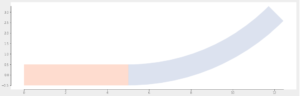#### Define custom pin positions

The new cell “myCell” has all connectivity attributes it needs and can be put. However, if it is put in the present from twice like

```C.put()
C.put()```

the result in gds will be two instances of ‘”myCell” in parent cell “Nazca”, but on top of each other, rather than chain connecting head to tail. Actually, it is chain connected, because ‘a0’ of the second C.put connects to ‘b0’ of the first put, but the default ‘a0’ and ‘b0’ are located in (x, y) = (0, 0). Hence, it makes sense to reposition ‘a0’ and ‘b0’ when creating the cell. This is done by creating a Nazca “Pin” object and putting it in the cell. The Pin object can be placed anyware in the cell, but here a logical place of ‘a0’ is at the input of the straight guide and for ‘b0’ at the output of the bent guide. Nazca allows for placing those pins by reference using the “pin” keyword in the Pin object. When designing a cell make sure pins are always outward pointing from a mask element to keep connections intuitive and uniform:

```# example created by Bright Photonics

import nazca as nd

with nd.Cell('myCell') as C:
C.autobbox = True
e1 = nd.strt(length=5, layer=1).put()
e2 = nd.bend(angle=45, layer=2).put()
nd.Pin(name='a0', pin=e1.pin['a0']).put()
nd.Pin(name='b0', pin=e2.pin['b0']).put()

C.put()
C.put()

for name, node in C.pin.items():
print(f"{name} @ {node.fxya()}")
# org @ (0.000, 0.000, 0.000)
# a0 @ (0.000, 0.000, 180.000)
# b0 @ (12.071, 2.929, 45.000)

nd.export_gds()```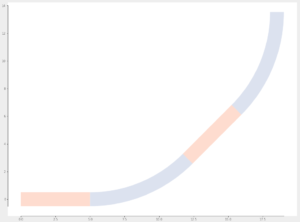#### Stubs (visualize pins)

It is possible to visualize the pins in the cell in gds export via Nazca stubs, as shown in the next example. The pins are by default indicated by an arrow shape, where the pin is located at the tip of the arrow and points in the direction of the arrow. The name of the pin is added to the gds as an gds annotation. Note that the ‘org’ pin is not visualized.

```# example created by Bright Photonics

import nazca as nd

with nd.Cell('myCell') as C:
e1 = nd.strt(length=5).put()
e2 = nd.bend(angle=45).put()
nd.Pin(name='a0', pin=e1.pin['a0']).put()
nd.Pin(name='b0', pin=e2.pin['b0']).put()
nd.put_stub()

C.put()

nd.export_gds()```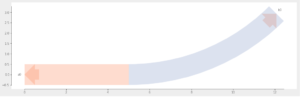A bounding box is added to the cell by setting the cell attribute autobbox = True. The bbox adds pins lb, lc, lt, tl, tc, tr, rt, rc, rb, br, bc, bl and cc. The letters indicate left, right, top and center. The first letter indicates the direction of the pin. The cc pin sits in the geometrical center of the bbox, but is not visualized:

```# example created by Bright Photonics

import nazca as nd

with nd.Cell('myCell') as C:
C.autobbox = True
e1 = nd.strt(length=5).put()
e2 = nd.bend(angle=45).put()
nd.Pin(name='a0', pin=e1.pin['a0']).put()
nd.Pin(name='b0', pin=e2.pin['b0']).put()
nd.put_stub()

C.put()

nd.export_gds()```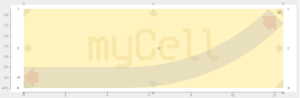#### Changing default pins and show some put options

A cell can have as many pins as needed, which can have any string name.  The default pin names can be customized as well, as demonstrated in the next code example. Note that the default input and output pin can be chosen to be the same pin. To show some options of the put function, a series of put statements have been added:

```# example created by Bright Photonics

import nazca as nd

with nd.Cell('myCell') as C:
C.default_pins('bert', 'ernie')
C.autobbox = True
e1 = nd.strt(length=5).put()
e2 = nd.bend(angle=45).put()
nd.Pin(name='bert', pin=e1.pin['a0']).put()
nd.Pin(name='ernie', pin=e2.pin['b0']).put()
nd.put_stub()

C.put()
C.put()
C.put(25) # x=25
C.put('bert', 25, 10) # x=25, y=10
C.put('bert', 25, 20, flip=True) # x=25, y=20
C.put('ernie', 25, 30, 0) # x=25, y=30, a=0

nd.export_gds()```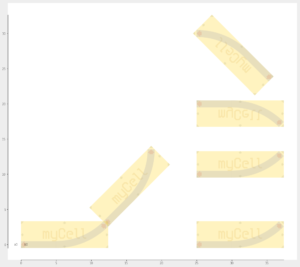## Related Tutorials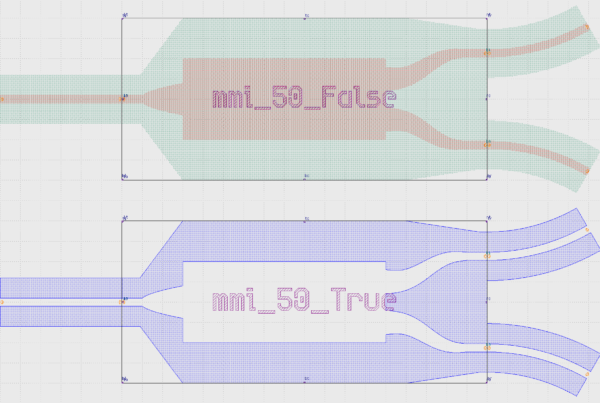In this example we show how to create an inverted MMI with custom interconnects.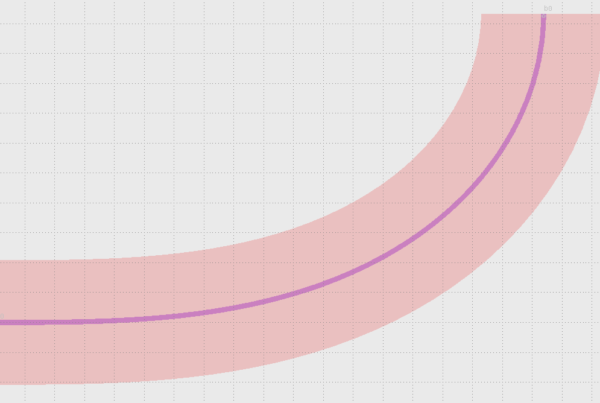In this example we show how to use Euler bends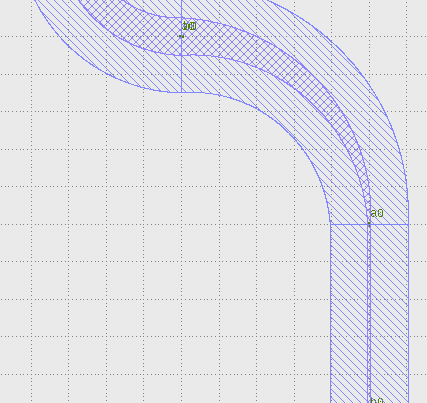In this example we show how to create a free form parametric curve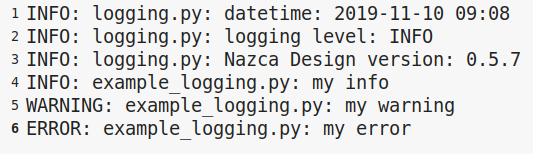In this example we show how to log your layout.